# Physical vs Logical Data Structure

## Physical vs Logical Data Structure:

In this article, we are just going to give an Introduction to Logical vs Physical Data structure. In our previous article, we discussed how a program utilized the main memory by dividing the memory into sections like stack and heap and we also discussed what is static and dynamic memory allocation. Data Structures are categorized as physical data structures and logical data structures.

##### Physical Data Structure:

The Array and LinkedList are the two physical data structures. We can have more physical data structures by taking the combination of these two data structures i.e. array and linked list.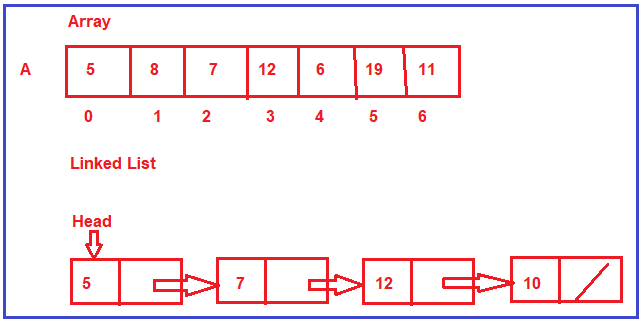##### Why we are calling array and linked list as physical data structures?

We call array and linked list as the physical data structure because these two data structures decide or define how the memory is organized or how the memory is allocated. So, let us look at them one by one

##### Array:

The Array is directly supported by programming languages such as C, C++, C#, Java, etc. The Array is a collection of contiguous memory locations i.e. all these locations are side by side. If we have an array for seven integers, then all these places for seven integers are together. They are in one place. The array will have a fixed size. Once it is created with some size, then that size cannot be increased or decreased. So, it is a fixed size i.e. the size of the array is static.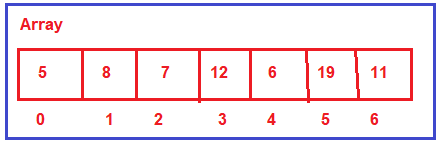##### Where the array can be created?

The array can be created either inside the stack or it can be created inside the heap. We can have a pointer, pointing to the array created on the heap. So, the array can be created either inside the stack or inside the heap.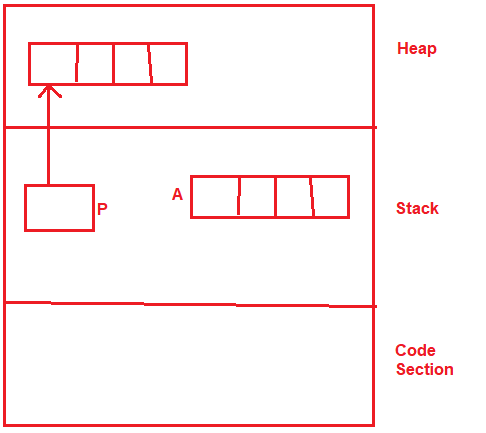##### When to use the array data structure?

We need to use the array data structure when we are sure what is the maximum number of elements that we are going to store. That means if we know the length of the list then we need to go for the array data structure.

The Linked List data structure is a completely dynamic data structure. It is a collection of nodes where each node contains data and a link to the next node. The length of the linked list can grow and reduce dynamically. So, it is having a variable length. As per our requirement, you can go on and adding more and more nodes and add more elements or you can reduce the size.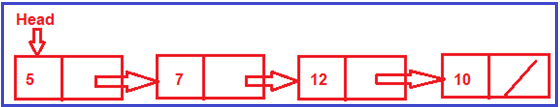##### Where the linked list can be created?

The linked list is always created in the heap i.e. collection of nodes is created always in heap and can be accessed using a pointer and that pointer is created inside the stack. So, a linked list is always created in the heap.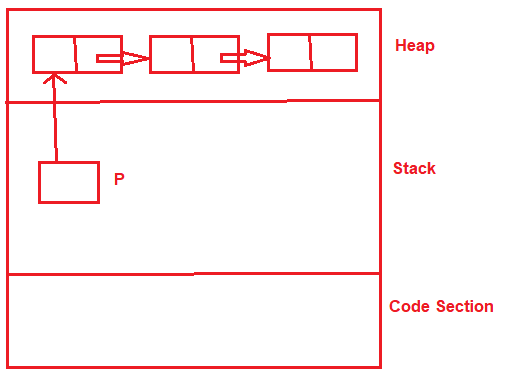##### When to use the Linked List data structure?

We need to go with the linked list data structure when we are not sure what is the maximum number of elements that we are going to store. That means if we don’t know the length of the list then we need to go for the linked list data structure.

These two data structures are physical data structures because they define how the memory should be organized for storing the elements or data. These are more related to memory. I have just introduced these two data structures as there are separate topics in our course for array and linked list where we will discuss array and linked list in detail. Now, let us move on to the next type of data structure that is the Logical Data Structure.

##### Logical Data Structure:

The following is a list of logical data structures.

1. Stack
2. Queue
3. Tree
4. Graph
5. Hash Table

Note: We will discuss each of the above logical data structures in detail in their respective chapter.

##### Difference between the logical data structure and the physical data structure:

Physical data structures are actually meant for storing the data in the memory. Then on the stored data or values, we may be performing some operations like inserting more values or deleting existing values or searching for the values, and many more operations. Now, the question is, how you want to utilize those values? How you will be performing insertion and deletion? What is the discipline that you are going to follow? That discipline is defined by logical data structures i.e. stack, queues, trees, graphs, and hash table.

Stack and Queue are Linear Data Structure. Tress and Graphs are non-linear data structures. The Hash table may be a linear or tabular data structure.

Stack works on the discipline of LIFO i.e. Last in First Out. Queue works on the discipline of FIFO i.e. First in First Out. The trees are a non-linear data structure and they will be organized in a hierarchy. The graph is a collection of nodes and the links between the nodes. These data structures are actually used in applications and algorithms.

The most important point that you need to remember is for implementing the logical data structures (Stack, Queue, Trees, Graphs, Hash Tables) we either use an array or linked list or a combination of array and linked list physical data structures. So, that is all we have given the introduction of various types of data structures. This was just the introduction to give us awareness.

##### Types of Data Structure:

Data Structure classified mainly into two types.

1. Linear Data Structure: A Linear data structure have data elements arranged in a sequential manner and each member element is connected to its previous and next element. Example: LinkedList, stacks, queue, etc.
2. Non-Linear Data Structures: A non-linear data structure has no set sequence of connecting all its elements and each element can have multiple paths to connect to other elements. Example: Trees, Graphs.

For a better understanding of the classification of data structure please have a look at the following diagram.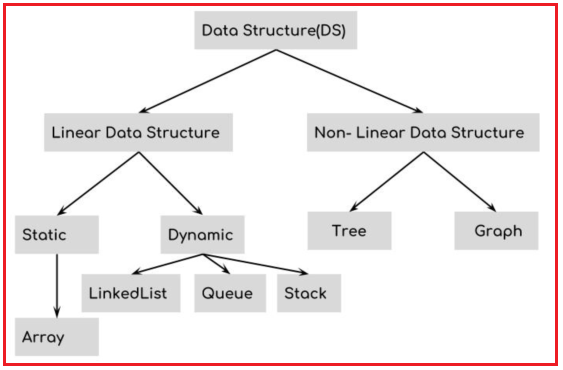The conclusion of this article is, we wanted to differentiate different types of data structures that are the physical data structure (arrays and linked lists) and logical data structures (stack, queue, trees, graphs, and hash table). The logical data structure is implemented using the physical data structures either using array and linked lists.

In the next article, I am going to discuss Stack vs Heap Memory in detail. Here, in this article, I try to give a brief introduction to Logical vs Physical Data structure and I hope you enjoy this Logical vs Physical Data structure article. I would like to have your feedback. Please post your feedback, question, or comments about this Introduction to Logical vs Physical Data structure article.

### 1 thought on “Physical vs Logical Data Structure”

1.Again Very Imformative first and Best 🙂